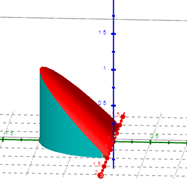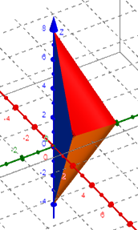# Plotting a solid which is bounded by several surfaces

Janet Napoles shared this question 2 years ago

Hello! Is there a way I can plot these? I'm having a hard time setting the bounds of the surfaces to just be below/above the intersections.1

For the second photo, the planes are z=3x=y-4 and z=8-3x-2y.1

xmin=-1

xmax=1

ymin=0

ymax=-sqrt(1-x^2)

zmin=0

zmax=-y1

Thank you!1

xmin=0

xmax=2

ymin=0

ymax=4-2x

zmin=3x + y - 4

zmax=8 - 3x - 2y1

also

0<-->pi ; 0<-->1 ; 0<-->rho sin(alpha)